# Static Games of Incomplete Information Mechanism design Typically

• Slides: 24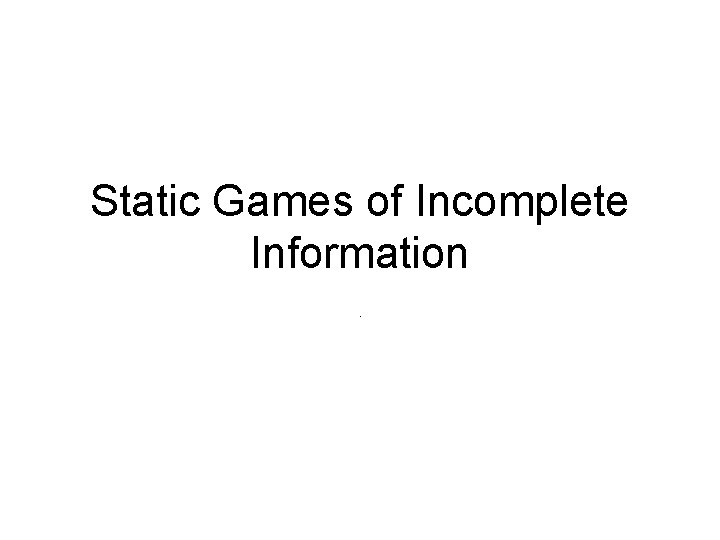Static Games of Incomplete Information.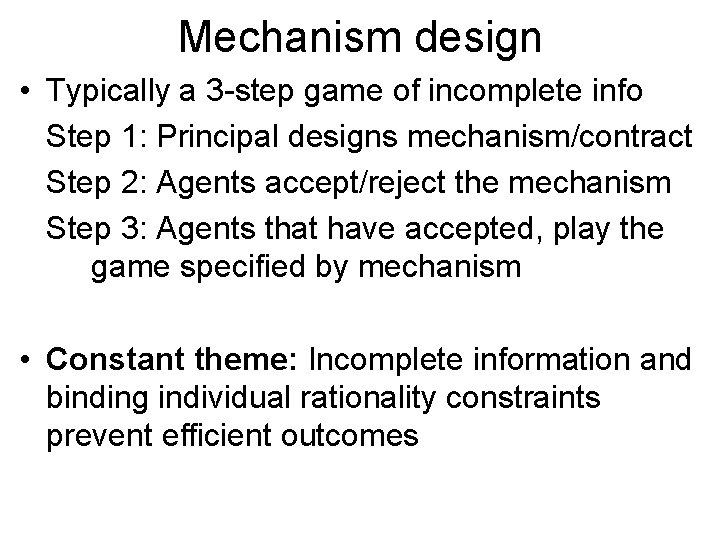Mechanism design • Typically a 3 -step game of incomplete info Step 1: Principal designs mechanism/contract Step 2: Agents accept/reject the mechanism Step 3: Agents that have accepted, play the game specified by mechanism • Constant theme: Incomplete information and binding individual rationality constraints prevent efficient outcomesNonlinear pricing • A monopolist produces good at marginal cost c and sells quantity q • Consumer transfers T to seller and has utility u 1(q, T, θ)= θV(q)-T, V(0)=0, V/>0, V//<0 • θ is private knowledge for buyer • Seller knows that θ= w. p. and θ= w. p. • The game: 1. Seller offers tariff T(q): specifies a price for qty q 2. Consumer accepts/rejects • If seller knows θ, she will charge T= θV(q), her profit, θV(q)-cq. This is maximized at some q given by θV/(q)=c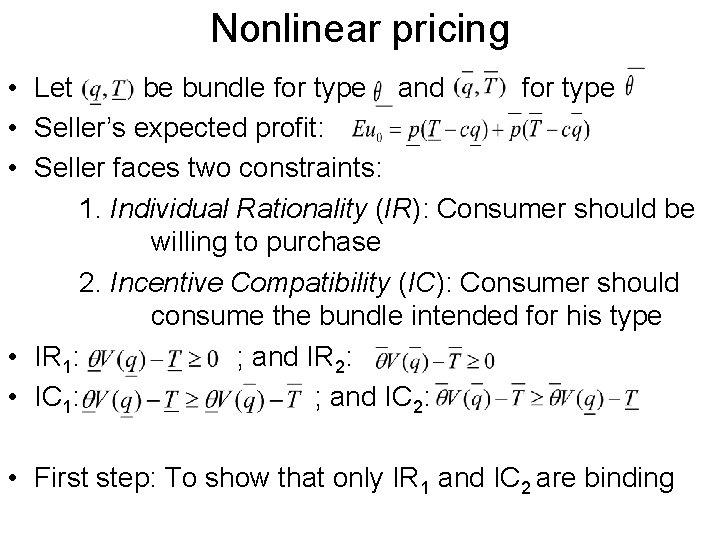Nonlinear pricing • Let be bundle for type and for type • Seller’s expected profit: • Seller faces two constraints: 1. Individual Rationality (IR): Consumer should be willing to purchase 2. Incentive Compatibility (IC): Consumer should consume the bundle intended for his type • IR 1: ; and IR 2: • IC 1: ; and IC 2: • First step: To show that only IR 1 and IC 2 are binding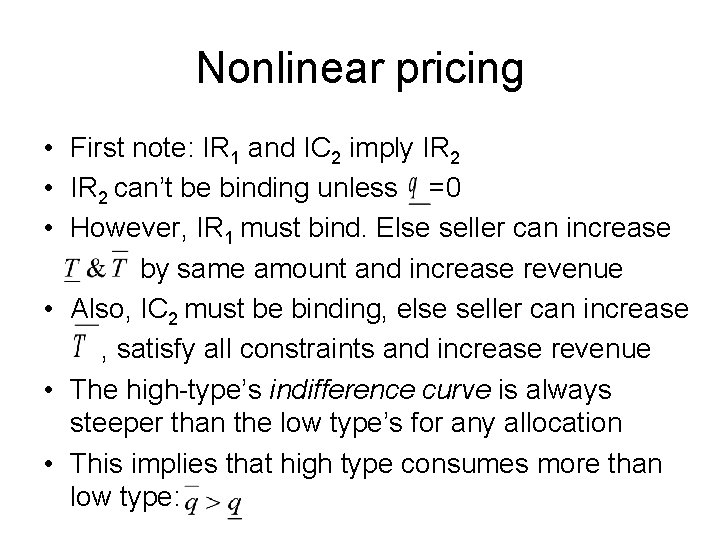Nonlinear pricing • First note: IR 1 and IC 2 imply IR 2 • IR 2 can’t be binding unless =0 • However, IR 1 must bind. Else seller can increase by same amount and increase revenue • Also, IC 2 must be binding, else seller can increase , satisfy all constraints and increase revenue • The high-type’s indifference curve is always steeper than the low type’s for any allocation • This implies that high type consumes more than low type: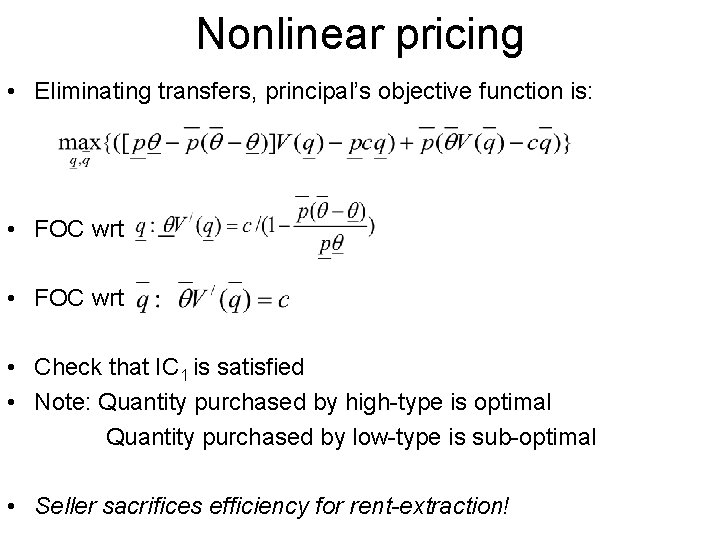Nonlinear pricing • Eliminating transfers, principal’s objective function is: • FOC wrt • Check that IC 1 is satisfied • Note: Quantity purchased by high-type is optimal Quantity purchased by low-type is sub-optimal • Seller sacrifices efficiency for rent-extraction!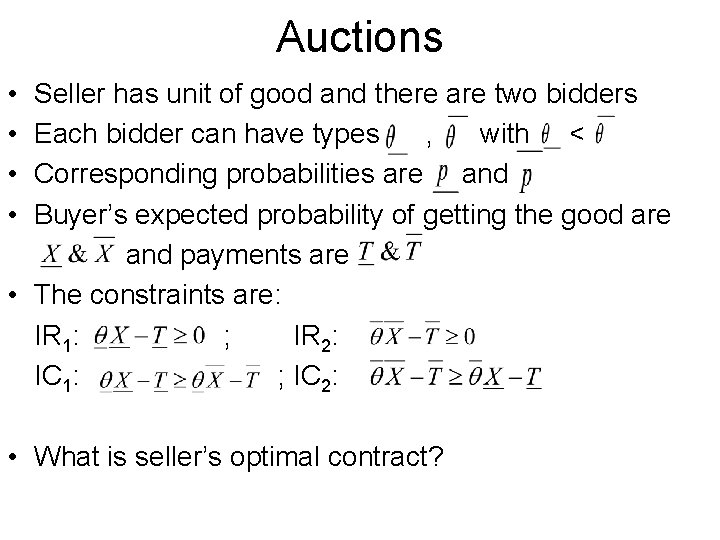Auctions • • Seller has unit of good and there are two bidders Each bidder can have types , with < Corresponding probabilities are and Buyer’s expected probability of getting the good are and payments are • The constraints are: IR 1: ; IR 2: IC 1: ; IC 2: • What is seller’s optimal contract?Auctions • Seller’s expected profit is: • Again, IR 1 and IC 2 are binding. The seller’s profit: • Also, ex-ante prob of a player getting good, • Moreover, • Case 1: . The seller sets and Optimal mechanism: Not to sell if both announce low-type; sell to high-type if they announce different types; sell wp ½ to each if both announce high type • Case 2: . The seller sets and Optimal mechanism: Sell to high-type if bidders announce different types, and sell wp ½ to each if they both announce high-type or low-type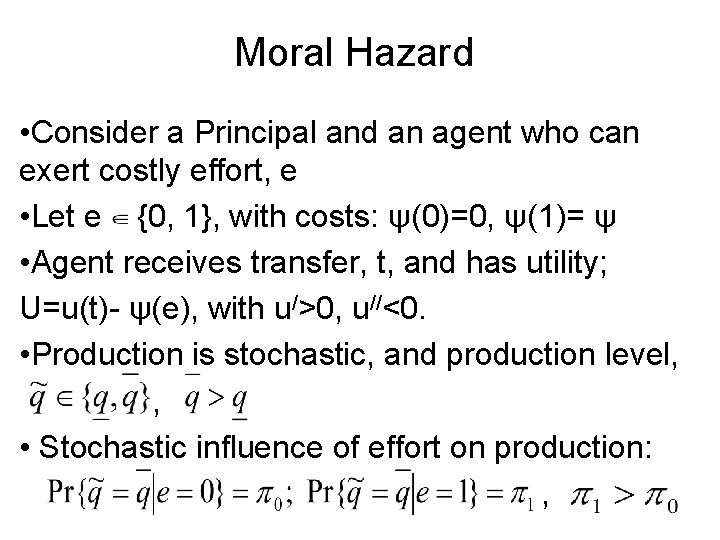Moral Hazard • Consider a Principal and an agent who can exert costly effort, e • Let e {0, 1}, with costs: ψ(0)=0, ψ(1)= ψ • Agent receives transfer, t, and has utility; U=u(t)- ψ(e), with u/>0, u//<0. • Production is stochastic, and production level, , • Stochastic influence of effort on production: ,Moral Hazard • Principal can offer a contract, {t( )}, that depends on observed, random output • With two possible outcomes, contract is: if output is and if output is • Let Principal’s profit with qty q be S(q) • His profit when agent expends effort e=0 is: • His profit when agent expends effort e=1 is: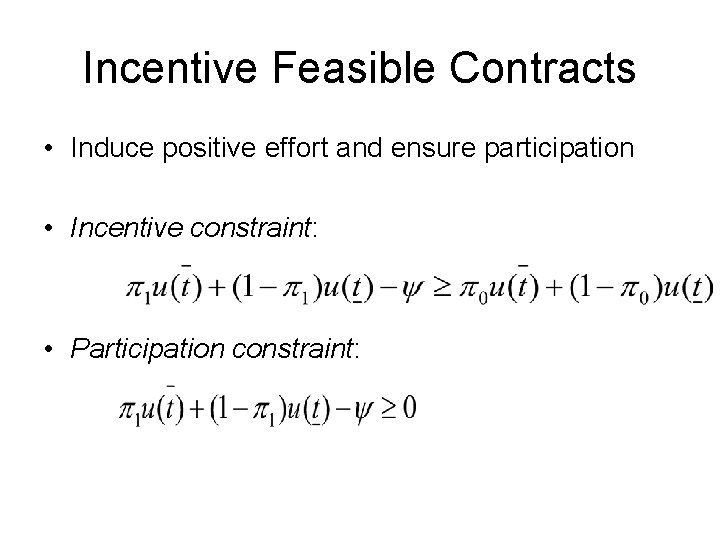Incentive Feasible Contracts • Induce positive effort and ensure participation • Incentive constraint: • Participation constraint: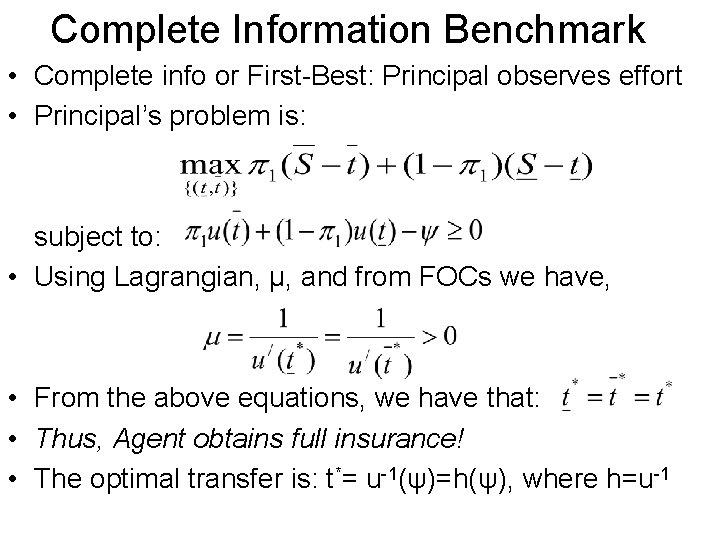Complete Information Benchmark • Complete info or First-Best: Principal observes effort • Principal’s problem is: subject to: • Using Lagrangian, μ, and from FOCs we have, • From the above equations, we have that: • Thus, Agent obtains full insurance! • The optimal transfer is: t*= u-1(ψ)=h(ψ), where h=u-1First Best Case • When there is complete information • Principal’s profit from inducing effort e=1: V 1= • If agent exerted 0 effort, principal would earn: V 0= • Inducing effort is optimal for principal if: , where • Principal’s First-Best cost of inducing effort is: h(ψ)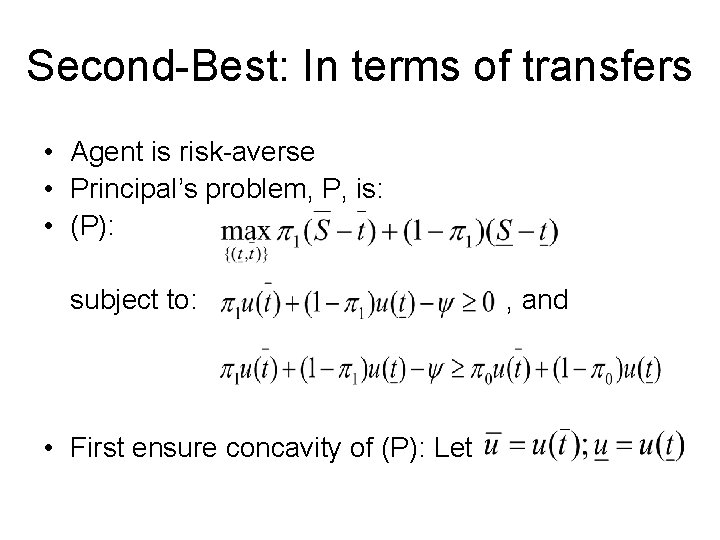Second-Best: In terms of transfers • Agent is risk-averse • Principal’s problem, P, is: • (P): subject to: • First ensure concavity of (P): Let , and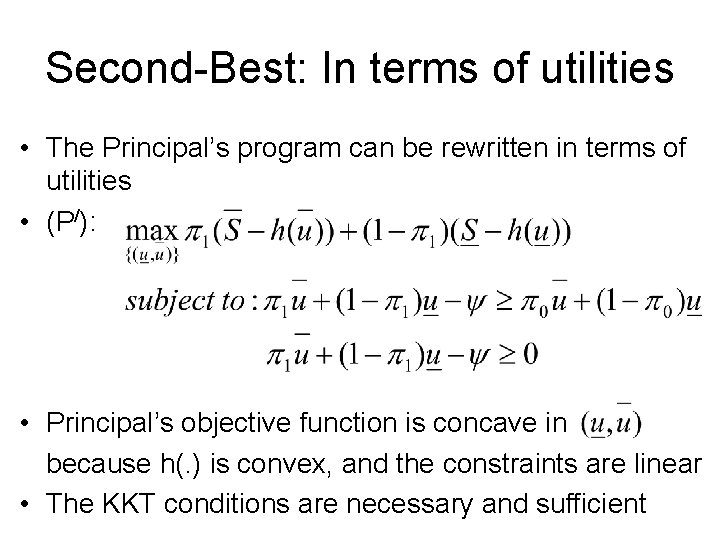Second-Best: In terms of utilities • The Principal’s program can be rewritten in terms of utilities • (P/): • Principal’s objective function is concave in because h(. ) is convex, and the constraints are linear • The KKT conditions are necessary and sufficient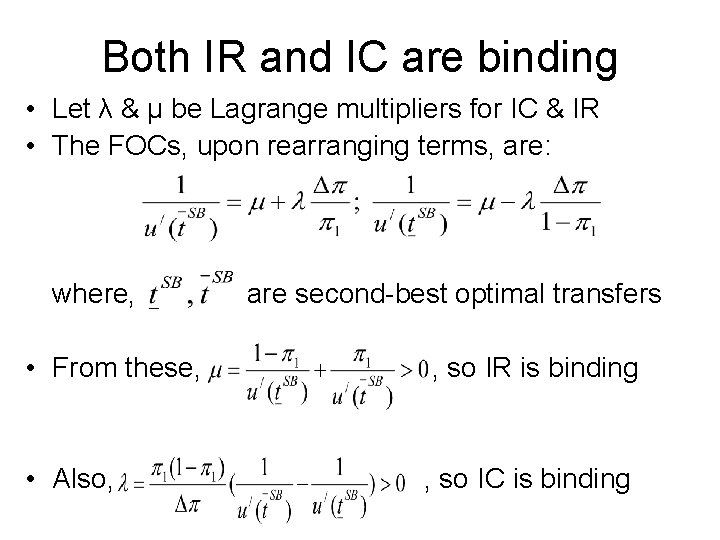Both IR and IC are binding • Let λ & μ be Lagrange multipliers for IC & IR • The FOCs, upon rearranging terms, are: where, are second-best optimal transfers • From these, , so IR is binding • Also, , so IC is bindingSecond-Best Solution • The variables ( , λ, μ ) are solved simultaneously from two FOCs, IC and IR • The second-best optimal transfers are: • : contract does not provide full insurance • 2 nd Best cost of inducing effort: CSB= • Clearly, for the Principal, CSB> CFB. So Principal induces high effort (e=1) less often than in first-best • There is under-provision of effort in the second-best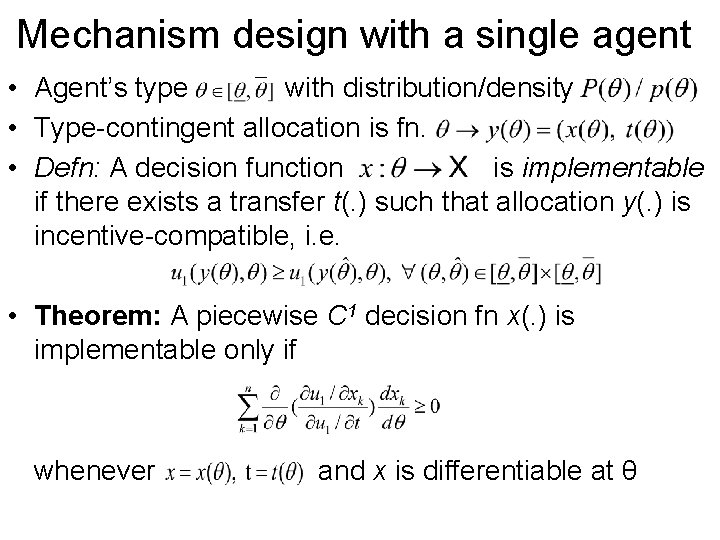Mechanism design with a single agent • Agent’s type with distribution/density • Type-contingent allocation is fn. • Defn: A decision function is implementable if there exists a transfer t(. ) such that allocation y(. ) is incentive-compatible, i. e. • Theorem: A piecewise C 1 decision fn x(. ) is implementable only if whenever and x is differentiable at θ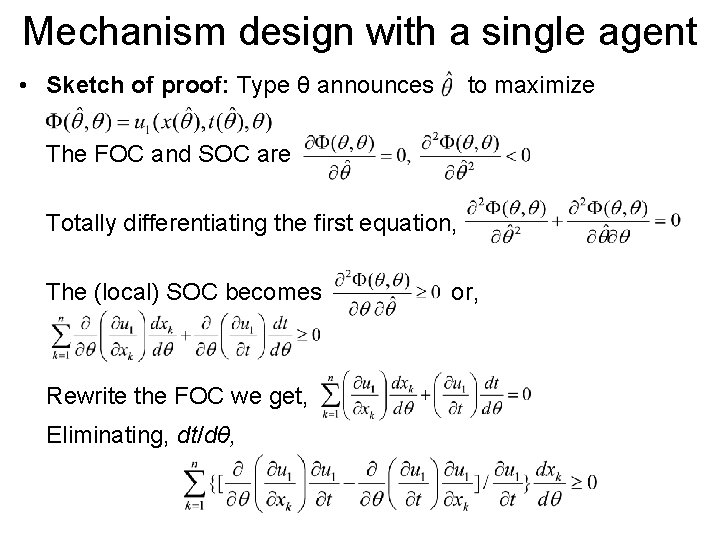Mechanism design with a single agent • Sketch of proof: Type θ announces to maximize The FOC and SOC are Totally differentiating the first equation, The (local) SOC becomes Rewrite the FOC we get, Eliminating, dt/dθ, or,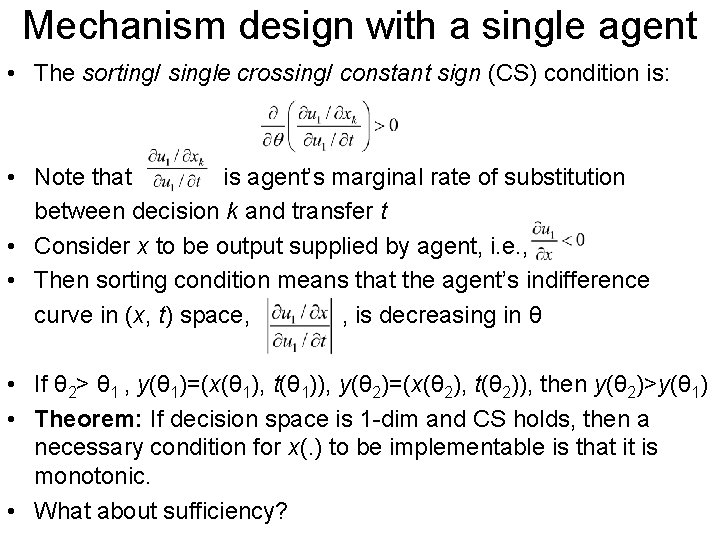Mechanism design with a single agent • The sorting/ single crossing/ constant sign (CS) condition is: • Note that is agent’s marginal rate of substitution between decision k and transfer t • Consider x to be output supplied by agent, i. e. , • Then sorting condition means that the agent’s indifference curve in (x, t) space, , is decreasing in θ • If θ 2> θ 1 , y(θ 1)=(x(θ 1), t(θ 1)), y(θ 2)=(x(θ 2), t(θ 2)), then y(θ 2)>y(θ 1) • Theorem: If decision space is 1 -dim and CS holds, then a necessary condition for x(. ) to be implementable is that it is monotonic. • What about sufficiency?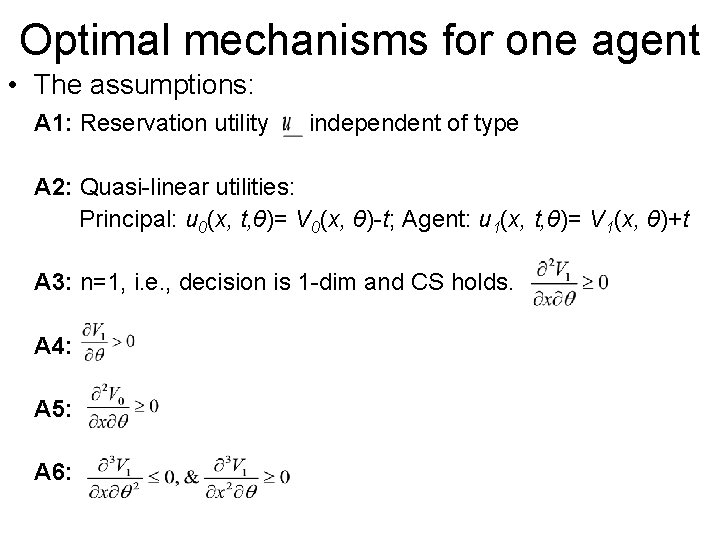Optimal mechanisms for one agent • The assumptions: A 1: Reservation utility independent of type A 2: Quasi-linear utilities: Principal: u 0(x, t, θ)= V 0(x, θ)-t; Agent: u 1(x, t, θ)= V 1(x, θ)+t A 3: n=1, i. e. , decision is 1 -dim and CS holds. A 4: A 5: A 6: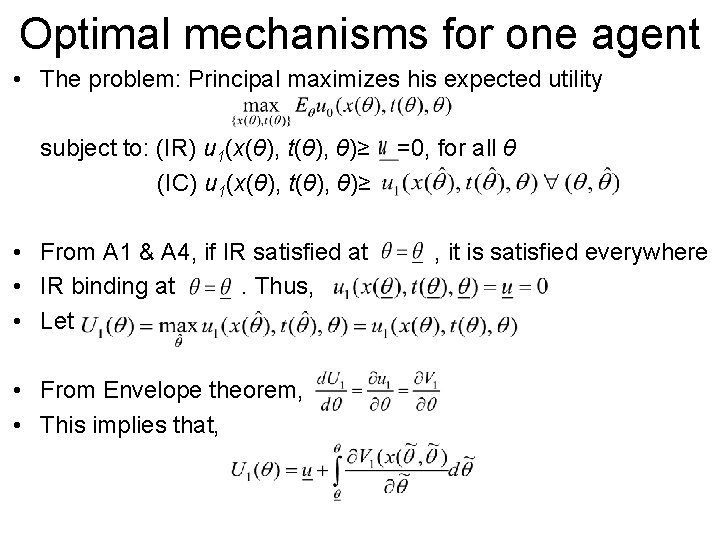Optimal mechanisms for one agent • The problem: Principal maximizes his expected utility subject to: (IR) u 1(x(θ), t(θ), θ)≥ (IC) u 1(x(θ), t(θ), θ)≥ • From A 1 & A 4, if IR satisfied at • IR binding at. Thus, • Let • From Envelope theorem, • This implies that, =0, for all θ , it is satisfied everywhereOptimal mechanisms for one agent • Further, u 0= V 0+ V 1 - U 1≡ Social surplus-Agent’s utility • Principal’s objective function: • Since monotonicity is necessary and sufficient for implementability, Principal’s optimization program becomes s. t. x(. ) is monotonic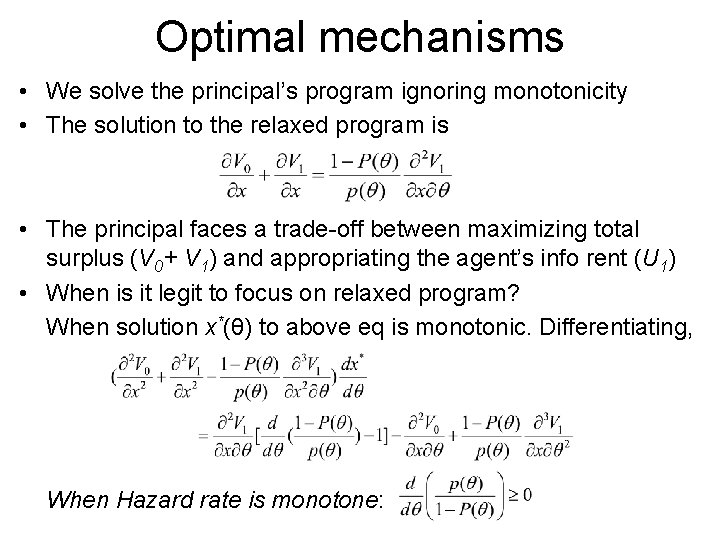Optimal mechanisms • We solve the principal’s program ignoring monotonicity • The solution to the relaxed program is • The principal faces a trade-off between maximizing total surplus (V 0+ V 1) and appropriating the agent’s info rent (U 1) • When is it legit to focus on relaxed program? When solution x*(θ) to above eq is monotonic. Differentiating, When Hazard rate is monotone: